Script Library: 1238 scripts

# Documentation for: r3d2.r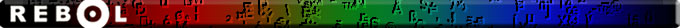# R3D Documentation

Massimiliano Vessi

## Contents:

1. Introduction
1.1 Test it
2. How it works
2.1 Basic principle
2.2 Basic usage
2.3 Specify objects position and rotation
2.4 Creating models
2.6 Going deep, to know all
3. Object File Format (.off)
4. All function descriptions
5. Models
6. Thanks

## 1. Introduction

R3D is a library to create and visualize 3D objects. It works on any platform (Windows, Linux or MacOS), because it use only DRAW. In order to use it, just copy r3d2.r in your folder script and add:

```do %r3d2.r
```

The main function is render, but there are many other useful functions. If you read the script, the first part contains just functions to operate with matrices 4x4 and 3x1. The second part of the script contains the more interesting functions.

It can also load OFF (object file format) of 3D objects.

If you want to correct or improve library or documentation, send me an email: maxint@tiscali.it

### 1.1 Test it

Try this and see the result:

```do http://www.rebol.org/download-a-script.r?script-name=advanced-r3d.r
```

result could be this: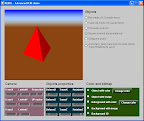a video about the demo is here: http://youtu.be/UL9Xb-fQX4U

## 2. How it works

3D is a complicated matter and to really understand deeply how all it works, you should study geometry and linear algebra. However I'll explain just how to use and basic principles of this library.

### 2.1 Basic principle

The basic principles are the followings:

• You create a word with 3D objects
• You put your camera int the the 3D word, giving position of the camera and the point you are looking. This is a very important concept, it is not enough to give camera position. Moreover if you move your camera, it will continue to look at the given point. This is different from human habit, if you walk on the street, you continue to look at 3 meters in front of you. Vice versa the camera, if you don't update also the point you are looking at, it will walk on the street starting looking at the traffic light in front of it; when it'll surpass the traffic light, it will turn back continuing to look at the traffic light, even it can't see the traffic light anymore. So remember to update also the point where the camera is looking when you move it.
• You must give the UP direction. Did you ever notice what happen rotating a camera during recording? Yes, the same way; you must give where is up and down, probably sometime Z axis is not a convenient UP.

### 2.2 Basic usage

```do %r3d2.r
```

First of all we create our world:

```world: copy []
```

Now we must create (or populate) a world using the cube2-model, every object you put in your world must have:

• model (cube, pyramid, an airplane, ...)
• proportion matrix: this is useful, for example a cube (a dice) can be deformed in a parallelepiped (a tower, a coffin, ...)
• color or image (color or image on the faces)

the proportion matrix is a 4x4 transformation matrix, the quick way is to use r3d-scale function and give the 3 size (X,Y,Z). We will use the red color so our word will be:

```model: reduce [cube2-model  (r3d-scale 100 100 400 )    red ]
```

now we insert our model in our world:

```append world reduce [model]
```

This way we create a red cube stretched on the Z axis four times that in the other direction (a tower).

Note

R3D contains "ready to use" models, in the next chapter will see how to create our model, now we will use cube2-model.

We can add many objects in our word, but in this first example we'll see only an object. The way used to populate the world is the one correct, since we can add many 3D objects in our world, like:

```world1: [ [only one object] ]
world2: [ [object1]  [object2]  ]
```

#### 2.2.2 Installing the camera

This is a very important concept: what will you show of your world?

You just created a world, but on a screen you have a 2D window, so you must put in your word a camera that look and show your word on the window. The camera is just a point, it doesn't have volume, it doesn't occupy space. So you must give:

• camera position
• point that is looking the camera
• UP vector (a 3x1 vector giving where is up)

Since the tower is height 100x100x400 (cube2-model is just 1x1x1 size), we could put our camera at point (300,300,600) looking to poit (0,0,0), considering the Z axis as UP. So our code will be:

```camera: r3d-position-object [ 300 300 600] [ 0 0 0] [ 0 0 1]
```

We must supply as camera a 4x4 camera projection matrix, the quickest way is to use the r3d-position-object function. Modifying the UP position, you'll obtain cool rotation.

#### 2.2.3 Showing all

Now we must decide our window size (for example 300x200) and decide the perspective projection matrix. Don't be scared of these terms, if you don't need some distortion, just use the r3d-perspective giving it a number greater than zero (for example 250 is a good number here).

So we put all together and send it to the render function:

```my-window: render world camera (r3d-perspective 250) 300x200
```

Now we can show all in our layout:

```view layout [
box 300x200 effect [draw my-window]
]
```

The result should be this: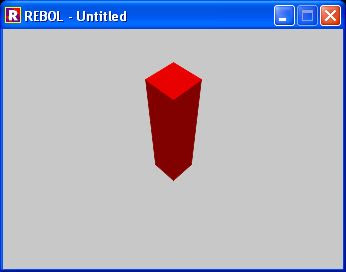#### 2.2.4 Some consideration

You'll notice that all the code to render a 3D world is extremely short and complete at the same time, all the code is

```do %r3d2.r
model: reduce [cube2-model  (r3d-scale 100 100 400 ) red ]
world: copy []
append world reduce [model]
camera: r3d-position-object [ 300 300 600] [ 0 0 0] [ 0 0 1]
my-window: render world camera (r3d-perspective 250) 300x200
view layout [ box 300x200 effect [draw my-window]  ]
```

No more than 7 lines.

You can play and examine the advanced-r3d.r script to learn how to play with R3D parameters.

### 2.3 Specify objects position and rotation

The proportion matrix can be of proportion, translation or rotation using the r3d-compose-m4 function. Let's see a dimostration:

```do %r3d2.r
model: reduce [cube2-model  (r3d-scale 100 100 400 ) red ]
world: copy []
append world reduce [model]
properties: r3d-compose-m4 reduce [r3d-scale 100 100 400  r3d-translate -110 110 0]
model2: reduce [cube2-model properties  red ]
append world reduce [model2]
camera: r3d-position-object [ 300 300 600] [ 0 0 0] [ 0 0 1]
my-window: render world camera (r3d-perspective 250) 300x200
view layout [ box 300x200 effect [draw my-window] ]
```

You'll obtain this: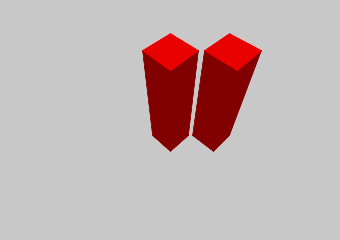### 2.4 Creating models

Models are 3D objects, they are represented by vertexes and faces. For example a cube is made of 8 vertexes and six faces. A model is made of block containing two blocks:

• vertexes coordinates
• faces made of vertexes, every block is a list of vertexes for that face

Attention: faces have only one side: the front; the order of the vertexes determines the front or the back of the face.

Example:

```cube2-model: [
; vertexes
[
[ 0 0 0 ] ;vertex 1
[ 1 0 0 ] ;vertex 2
[ 1 1 0 ] ;vertex 3
[ 0 1 0 ] ; vertex 4
[ 0 0 1 ] ;point 5
[ 1 0 1 ] ;point 6
[ 1 1 1 ] ;point 7 and so on...
[ 0 1 1 ]
]
; faces - anticlockwise winding
[
[ 4 3 2 1] ;bottom face made of vertexes 1 2 3 4
[ 5 6 7 8 ] ; upper face
[ 1 5 8 4 ]
[ 1 2 6 5]
[ 2 3 7 6 ]
[ 8 7 3 4  ]
]
]
```

You can describe a face with a minimum of 3 points (triangular face). As you can see you can determine a face with any number of vertex, not only triangle (as other 3D programs). Look at this model:

```prysm-8-model: [
;vertices
[
[-0.414 -1 0] ;1
[0.414 -1 0] ;2
[1 -0.414 0 ] ;3
[1 0.414 0 ] ;4
[0.414  1 0] ;5
[-0.414  1 0] ;6
[-1 0.414 0 ] ;7
[-1 -0.414 0 ] ;8
[-0.414 -1 1] ;9
[0.414 -1 1] ;10
[1 -0.414 1 ] ;11
[1 0.414 1 ] ;12
[0.414  1 1] ;13
[-0.414  1 1] ;14
[-1 0.414 1 ] ;15
[-1 -0.414 1 ] ;16
]
; faces
[
[1 2   3   4   5   6   7   8]
[9 10 11 12 13 14 15 16]
[1 2 10 9]
[2 3 11 10]
[3 4 12 11]
[4 5 13 12]
[5 6 14 13]
[6 7 15 14]
[7 8 16 15]
[8 1 9 16]
]
]
```

It's an octagonal base prysm, you can describe it with just 10 faces: 2 octagons + 8 rectangles! Other old 3D techniques use only triangles, so you had to specify 32 faces. Rebol is the next generation computer graphic! Using the following code:

```model: reduce [prysm-8-model  (r3d-scale 100 100 200 ) red ]
world: copy []
append world reduce [model]
camera: r3d-position-object [ 300 300 400] [ 0 0 0] [ 0 0 1]
my-window: render world camera (r3d-perspective 250) 300x200
view layout [ box 300x200 effect [draw my-window]]
```

this is the result: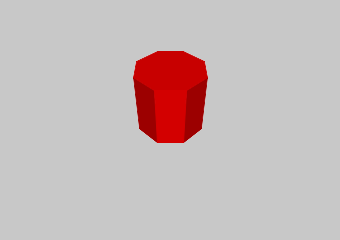If you specify an image as color, you may obtain two different results:

If the face is rectangular (made of exactly 4 vertexes), you have the image attached to the face, for example

```do %r3d2.r
model: reduce [cube2-model  (r3d-scale 200 200 200 )   img ]
world: copy []
append world reduce [model]
camera: r3d-position-object [ 300 300 300] [ 0 0 0] [ 0 0 1]
my-window: render world camera (r3d-perspective 250) 300x200
view layout [box 300x200 effect [draw my-window] ]
```

this is the result: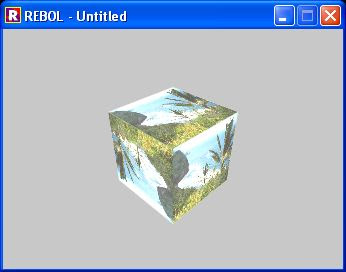if the face is not rectangular, you have a "transparent effect" of the image beyond the object. The image is repeated in order to fil the window background, for example cube-model is made of triangles, not of squares:

```do %r3d2.r
model: reduce [cube-model  (r3d-scale 200 200 200 )   img ]
world: copy []
append world reduce [model]
camera: r3d-position-object [ 300 300 300] [ 0 0 0] [ 0 0 1]
my-window: render world camera (r3d-perspective 250) 300x200
view layout [box 300x200 effect [draw my-window] ]
```

this is the result:### 2.6 Going deep, to know all

If you are interested in geometry and all calculus of the library, you can start reading the basic principles:

## 3. Object File Format (.off)

Object File Format (.off) files are used to represent the geometry of a model by specifying the polygons of the model's surface. The polygons can have any number of vertices.

The .off files in the Princeton Shape Benchmark conform to the following standard. OFF files are all ASCII files beginning with the keyword OFF. The next line states the number of vertices, the number of faces, and the number of edges. The number of edges can be safely ignored.

The vertices are listed with x, y, z coordinates, written one per line. After the list of vertices, the faces are listed, with one face per line. For each face, the number of vertices is specified, followed by indices into the list of vertices. See the examples below.

Note that earlier versions of the model files had faces with -1 indices into the vertex list. That was due to an error in the conversion program and should be corrected now.

```OFF numVertices numFaces numEdges
x y z
x y z
... numVertices like above
NVertices v1 v2 v3 ... vN
MVertices v1 v2 v3 ... vM
... numFaces like above
```

Note that vertices are numbered starting at 0 (not starting at 1), and that numEdges will always be zero.

A simple example for a cube:

```OFF
8 6 0
-0.500000 -0.500000 0.500000
0.500000 -0.500000 0.500000
-0.500000 0.500000 0.500000
0.500000 0.500000 0.500000
-0.500000 0.500000 -0.500000
0.500000 0.500000 -0.500000
-0.500000 -0.500000 -0.500000
0.500000 -0.500000 -0.500000
4 0 1 3 2
4 2 3 5 4
4 4 5 7 6
4 6 7 1 0
4 1 7 5 3
4 6 0 2 4
```

Note

A lot of models on internet haven't a good face orientation, if you have some trouble to see them, there is the /no-cull refinement that you can use. Using it you'll see front and back of a face. Try it.

When you load a model, you don't know the scale, so r3d-Load-OFF function add a number ad the end, this is the suggested scale, you can add this code to yours:

```; calculate default scale from model/3 if it exists
modelsize: 1.0
modelsize: model/3
if modelsize < 1.0 [ modelsize: 1.0 ]
defaultScale: 512 / modelsize
dimx: dimy: dimz:  defaultScale
```

## 4. All function descriptions

 r3d-identity It's not a function, is just a 4x4 identity matrix (all 1 on the first diagonal). Useful for starting a matrix or resetting a matrix, and much more... r3d-perspective Create a perspective matrix with a vanishing point d units from the camera r3d-translate Create a translation matrix r3d-scale Create a scale matrix r3d-rotateX Create a rotation matrix around X axis r3d-rotateY Create a rotation matrix around Y axis r3d-rotateZ Create a rotation matrix around Z axis r3d-face-direction ???? r3d-position-object ??? r3d-m4xm4 Matrix product between two 4x4 matrices r3d-m4xv3 Matrix product between a 4x4 matrix and a 4x1 ([A B C 1]) vector. This function will append the last 1 to the vector 3x1 r3d-m4xv3-array This function return the same block of vertexes, multiplied for the transformation matrix r3d-compose-m4 Take a block containing 4x4 matrices and multiplicated each other obtaining a single 4x4 matrix r3d-transpose-m4 Transpose a 4x4 matrix r3d-inverse-m4 Inverse of a 4x4 matrix r3d-dotproduct Returns the dot product (a number) between two 3x1 vector r3d-crossproduct Returns a cross product (vector, 3x1) between 2 vectors (3x1) r3d-length Returns the distance between origin and a point (3x1) r3d-Add Add a to b - a and b can be either matrices or vectors but types must match r3d-Subtract Subtract b from a - a and b can be either matrices or vectors but types must match r3d-Multiply Multiply a by n - a and b can be either a matrix or vector, n is a number r3d-Divide Divide a by n - a and b can be either a matrix or vector, n is non-zero number r3d-normalise Normalize a vector, its module becomes 1 r3d-print-m4 Debug: Print a 4x4 matrix r3d-print-v3 Debug: Print a 3x1 vector render Main function that render 3D objects creating a VID DRAW r3d-CalculateFaceNormals Calculate face normals to apply light effects??? r3d-Render2dTriangles-Simple Decide which face to show and the light of the face??? r3d-Load-OFF Loads OFF files of 3D objects

## 5. Models

This is a list of the internal ready to use 3D objects:

 cube-model A cube made of triangle faces cube2-model A cube made of triangle faces, useful for images pyramid-model A triangular base pyramid, made of triangles square-pyramid-model A square base pyramid, made of triangles and a square wall-model A wall on the X plane, good for images ,remember faces have just one side. prysm-8-model A octagonal prism, it shows how to use polygons for faces.

## 6. Thanks

Thank to Andrew Hoadley to developed a such beautiful library.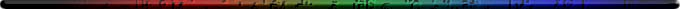MakeDoc2 by REBOL- 17-Oct-2012